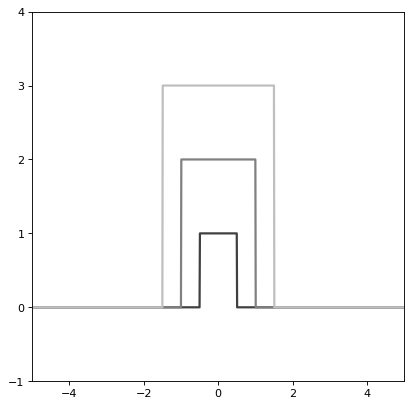# 方框1D¶

class astropy.modeling.functional_models.Box1D(amplitude=1, x_0=0, width=1, **kwargs)[源代码]

amplitude浮动

x_0浮动

width浮动

fixed口述，可选

tied可选的

bounds可选的

eqcons可选列表

ineqcons可选列表

$f（x）=\左\{$

import numpy as np
import matplotlib.pyplot as plt

from astropy.modeling.models import Box1D

plt.figure()
s1 = Box1D()
r = np.arange(-5, 5, .01)

for factor in range(1, 4):
s1.amplitude = factor
s1.width = factor
plt.plot(r, s1(r), color=str(0.25 * factor), lw=2)

plt.axis([-5, 5, -1, 4])
plt.show()


(png _, svgpdf此属性用于指示evaluate方法所需的单元或单元集，并返回将输入映射到单元（或 None 如果接受任何单位）。 描述此类型模型的参数的名称。 此属性用于指示evaluate的输出应包含哪些单元或单元集，并返回将输出映射到单元（或）的字典 None 如果接受任何单位）。

 evaluate 宽度，宽度 一维盒模型函数

amplitude = Parameter('amplitude', value=1.0)
input_units
param_names = ('amplitude', 'x_0', 'width')

return_units
width = Parameter('width', value=1.0)
x_0 = Parameter('x_0', value=0.0)

static evaluate(x, amplitude, x_0, width)[源代码]